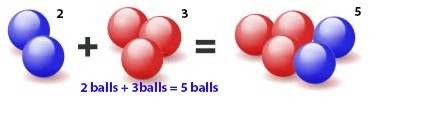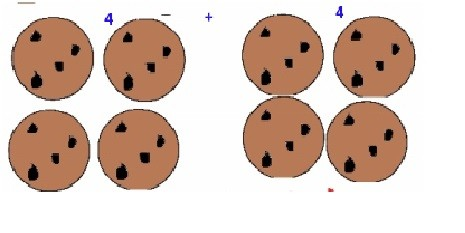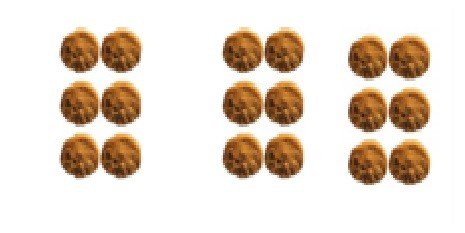What is basic mathematics?

Basic Mathematics is define as the “the science of Quantity” it gives you the basic concepts of mathematics. Every student must know these concepts and practice them in daily life through word problems.

Basic mathematics is the simple concepts, which makes student ready for learning fractions, LCM and GCF. Generally basic mathematics is Counting, Addition, Subtraction, Multiplication and Division. All the mathematical concepts are based on these four operations (Addition, Subtraction, Multiplication and Division). With these operations student also need to understand the different properties and relation with these operations.

Here are the basics mathematics general concepts along with examples for students who want to learn basic mathematics.Today we are going to discuss about kids Math,Which is very important to start learning mathematics.

## Counting

They are also called Natural numbers because they are naturally the first numbers we learn (1, 2, 3, 4 and so on). Sometimes they are also called as positive numbers.

These Arithmetic operations are used to count the physical objects in the world.

0, 1, 2, 3, 4, 5, 6, 7, 8, 9, 10…

EVERYDAY EXAMPLES

• `Count how many days left in this week?`

Answer: If today is Monday than 6 days left.

• `Count numbers from 1 to 20`

• Count socks to check that you have matching pairs (2).
`Answer: If you have 6 socks then you have 12 matching pairs.`
• Count how many people in your home.
• Count the students in your class.
• Count how many days left for your papers.
• Count the all alphabets. (A, B, C… so on).
• Put all these numbers in correct order (98, 23, 67, 12, 45, 56, and 10).

Your child knows counting if answers all the questions correctly. Although he/she needed is some practice daily.

Addition is the one of the basic operation of mathematics.  In simple words addition means joining two things together or adding two things. It can also define as the collection of objects or adding them together. It is represented by

“+” sign.

Examples

Let’s say you have 2 blue balls and your friend John has 3 red balls. You and John think that you can total the balls so that you both have more balls to play but first you find the total number of balls each one have.

Rather than counting the each ball separately, you can use basic mathematics operation (Addition) to find the total balls faster. Addition is where we put two numbers together to find the sum of the numbers.

You have 2 balls and John have 3 balls, so the sum of balls is 5.

2 blue balls + 3 red balls = 5 ballsExample2

Let’s say James has 4 cookies and John has 4 cookies. They want to make a cupcake but for that they have to total it how many cookies do they have.

Rather than counting individual cookies, just they use basic Arithmetic operation (total) for adding the numbers.Example

5             12              34                56                  47

+ 19          + 34            +14            +34                +23

___________________________________________________

Answers:                          24              46              48              90                  70

#### Subtraction

It’s a basic arithmetic operation use to find the difference between two numbers. When you have a lot of money and you subtract half of it then the money becomes smaller. There are three parts in subtraction the starting number is minuend. The number which is subtracted is subtrahend and the number which is left after subtraction is known as difference.in the below problem 5 is minuend 2 is subtracted and the answer 3 is difference.

Subtraction Methods

There are different methods of subtractions method one is to simply minus the two numbers as given below

Examples

If you have 5 eggs and you eat 2 eggs then how many eggs left?

5 eggs – 2 eggs = 3 eggs

Method 2: in daily life you can

Example:

Solve the examples by subtraction.

 Subtract A 12 34 23 13 7 3 67 _ B 2 32 12 10 3 1 34 A-B 10 2 11 3 4 2 33

#### Multiplication

You know it or not but sometimes you do multiplication just by adding them.

There are two methods of multiplication.

Method 1:

Example:In the above picture there are three groups and each of these groups has 6 cookies.

So the total cookies in three groups are 5 + 5 + 5=15 cookies.

Example 2:

Let’s say you have three groups and in each group there are 6 markers, so you count the total markers.

So             6 markers + 6 markers + 6 markers=18 markers.

Method 2:

Examples

 Multiplication A 12 6 5 9 4 3 4 * B 2 5 3 10 3 1 8 A * B 24 12 15 90 12 3 32

Example:

11         13         2          4          9             7       6

* 2        * 4         * 5        * 3         * 2         *7       * 2

__________________________________________________________

Answers                                   22       52       10           12      18        49           12

### Division

Division is the simply a process of divide a number into two equal parts. When you divide a number you start with large number after divide it split into its half. The dividend is the first number; the divisor is the number you want to split and the quotient is the answer of the number.

Dividend 10/ divisor 2= quotient 5

To represent division we have two operators

1. / Division slashes.
2. ÷ Division sign.

To divide the any numbers you always need these steps:

• Divide
• Multiply
• Subtract
• Drop number down

Repeating these steps until the problem is solved.

Cases:

There are some cases considered when dividing the two numbers.

• When we divide any number by one, the answer is always the original number.

20 / 1 =20                            5 / 1 =5

• Divisible by zero: When you divide a number by zero the answer is always undefined. In other words you can’t divide a number by zero.

20/0= undefined                40/0=undefined.

If dividend equal to divisor then the answer is always equal to one.

20/20 = 1                      2)   5/5 = 1

Example:

 Division A 12 6 12 10 20 3 8 / B 2 3 3 2 5 1 4 A / B 6 2 4 5 4 3 2